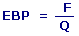Efficiency Bandwidth Product Calculators Enter value, select unit and click on calculate. Result will be displayed.

Calculate Efficiency Bandwidth Product (EBP)
Calculate Speaker Resonance Frequency
Calculate Speaker Electrical

 Web www.calculatoredge.com

Calculate Efficiency Bandwidth Product (EBP)EBP = Efficiency Bandwidth Product F = Speaker Resonance Frequency Q = Speaker Electrical
 Enter your values: Speaker Resonance Frequency: Hertz 1 / Second Cycle / Second Rotation / Second Speaker Electrical : Result: Efficiency Bandwidth Product:

Calculate Speaker Resonance Frequency

F = EBP x Q

 F = Speaker Resonance Frequency EBP = Efficiency Bandwidth Product Q = Speaker Electrical
 Enter your values: Efficiency Bandwidth Product: Speaker Electrical : Result: Speaker Resonance Frequency: Hertz

Calculate Speaker ElectricalQ = Speaker Electrical F = Speaker Resonance Frequency EBP = Efficiency Bandwidth Product
 Enter your values: Speaker Resonance Frequency : Hertz 1 / Second Cycle / Second Rotation / Second Efficiency Bandwidth Product : Result: Speaker Electrical :LINKSDISCLAIMERCONTACT US